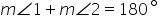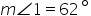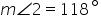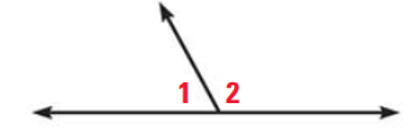Mathematics
Easy

Question

# The steps of the proof are shown.Given:PROVED:1. m ∠ 1 + m ∠ 2 = 180 (Given)2. m ∠ 1 = 62 (_____)3. 62 + m ∠ 2 = 180 (Substitution Property)4. m ∠ 2 = 118 (_____)What is the reason for step 2 and step 4?

## 2- Subtraction Property, 4- Subtraction Property2- Given, 4- Subtraction Property2- Distributive Property, 4- Substitution Property2- Given, 4- Substitution PropertyHint:

## The correct answer is: 2- Given, 4- Subtraction Property

### Here we have to find the reason for step 2 and step 4 . The given is m∠1 + m∠2 = 180° , And also m∠1 = 62°.Now , m∠1 + m∠2 = 180° ( given) m∠1 = 62° ( given) 62 + m∠2 = 180° ( substitution property ) m∠2 =118° ( subtraction property )Therefore, the reason of step 2 and step 4 is given and subtraction property.The correct answer is 2- Given , 4- Subtraction property.Or, simplyThe whole proof:m ∠ 1 + m ∠ 2 = 180 (Given)m ∠ 1 = 62 (Given)62 + m ∠ 2 = 180 (Substitution Property)m ∠ 2 = 180 - 62 = 118 (Subtraction Property)

In this question, we have to step 2 and step 4 reason . For that we must know the property or equality. Here step 2 is given but step 4 is subtraction property. In subtraction property, if A=B , then A – x = B – x .

### Related Questions to study#### With Turito Foundation.#### Get an Expert Advice From Turito.